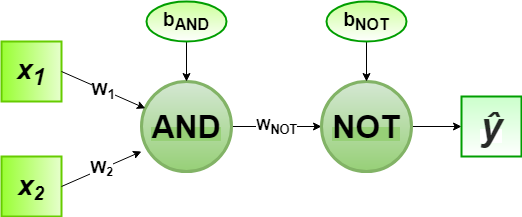Related Articles

# Implementation of Perceptron Algorithm for NAND Logic Gate with 2-bit Binary Input

• Last Updated : 08 Jul, 2020

In the field of Machine Learning, the Perceptron is a Supervised Learning Algorithm for binary classifiers. The Perceptron Model implements the following function:For a particular choice of the weight vectorand bias parameter, the model predicts outputfor the corresponding input vector.

NAND logical function truth table for 2-bit binary variables, i.e, the input vectorand the corresponding output001
011
101
110

We can observe that,Now for the corresponding weight vectorof the input vectorto the AND node, the associated Perceptron Function can be defined as:Later on, the output of AND nodeis the input to the NOT node with weight. Then the corresponding outputis the final output of the NAND logic function and the associated Perceptron Function can be defined as:For the implementation, considered weight parameters areand the bias parameters are.

Python Implementation:

 # importing Python libraryimport numpy as np  # define Unit Step Functiondef unitStep(v):    if v >= 0:        return 1    else:        return 0  # design Perceptron Modeldef perceptronModel(x, w, b):    v = np.dot(w, x) + b    y = unitStep(v)    return y  # NOT Logic Function# wNOT = -1, bNOT = 0.5def NOT_logicFunction(x):    wNOT = -1    bNOT = 0.5    return perceptronModel(x, wNOT, bNOT)  # AND Logic Function# w1 = 1, w2 = 1, bAND = -1.5def AND_logicFunction(x):    w = np.array([1, 1])    bAND = -1.5    return perceptronModel(x, w, bAND)  # NAND Logic Function# with AND and NOT  # function calls in sequencedef NAND_logicFunction(x):    output_AND = AND_logicFunction(x)    output_NOT = NOT_logicFunction(output_AND)    return output_NOT  # testing the Perceptron Modeltest1 = np.array([0, 1])test2 = np.array([1, 1])test3 = np.array([0, 0])test4 = np.array([1, 0])  print("NAND({}, {}) = {}".format(0, 1, NAND_logicFunction(test1)))print("NAND({}, {}) = {}".format(1, 1, NAND_logicFunction(test2)))print("NAND({}, {}) = {}".format(0, 0, NAND_logicFunction(test3)))print("NAND({}, {}) = {}".format(1, 0, NAND_logicFunction(test4)))
Output:
NAND(0, 1) = 1
NAND(1, 1) = 0
NAND(0, 0) = 1
NAND(1, 0) = 1


Here, the model predicted output () for each of the test inputs are exactly matched with the NAND logic gate conventional output () according to the truth table for 2-bit binary input.
Hence, it is verified that the perceptron algorithm for NAND logic gate is correctly implemented.

Attention reader! Don’t stop learning now. Get hold of all the important Machine Learning Concepts with the Machine Learning Foundation Course at a student-friendly price and become industry ready.

My Personal Notes arrow_drop_up